A Hierarchical Bayesian Model of the Premier League

Last fall, I was listening to an episode of the BS Report podcast in which Bill Simmons and Cousin Sal were discussing the strength of different NFL teams. It was early in the season - week 3 or 4, maybe - and Bill made the point (I'm paraphrasing here) that your estimate of one team's strength depends on your estimate of all the others. The conclusions you draw from team X beating team Y depends how strong team Y is, which in turn depends on the conclusions you draw from team Y's other games, which in turn depends on how strong Y's opponents were, etc.

It occurred to me that this problem is perfect for a Bayesian model. We want to infer the latent paremeters (every team's strength) that are generating the data we observe (the scorelines). Moreover, we know that the scorelines are a noisy measurement of team strength, so ideally, we want a model that makes it easy to quantify our uncertainty about the underlying strengths.

So I googled 'Bayesian football' and found this paper, called 'Bayesian hierarchical model for the prediction of football results.' The authors (Gianluca Baio and Marta A. Blangiardo) being Italian, though, the 'football' here is soccer.

In this post, I'm going to reproduce the first model described in the paper using pymc. While they used Seria A in their paper, I'm going to use the 2013-2014 Premier League.

Getting and cleaning the data¶

I got the data from wikipedia. I copied the 'Result Table' and saved it as a text file.

In :
%matplotlib inline
%config InlineBackend.figure_format = 'retina'
In :
import os
import math
import warnings
warnings.filterwarnings('ignore')

from IPython.display import Image, HTML
import numpy as np
import pandas as pd
import seaborn as sns
import matplotlib.pyplot as plt
import pymc # I know folks are switching to "as pm" but I'm just not there yet
In :
DATA_DIR = os.path.join(os.getcwd(), 'data/')
CHART_DIR = os.path.join(os.getcwd(), 'charts/')
In :
data_file = DATA_DIR + 'premier_league_13_14.txt'
Out:
ARS AST CAR CHE CPA EVE FUL HUL LIV MNC MNU NEW NOR SOT STO SUN SWA TOT WBA WHU
Home \ Away
Arsenal NaN 1-3 2-0 0-0 2-0 1-1 2-0 2-0 2-0 1-1 0-0 3-0 4-1 2-0 3-1 4-1 2-2 1-0 1-0 3-1
Aston Villa 1-2 NaN 2-0 1-0 0-1 0-2 1-2 3-1 0-1 3-2 0-3 1-2 4-1 0-0 1-4 0-0 1-1 0-2 4-3 0-2
Cardiff City 0-3 0-0 NaN 1-2 0-3 0-0 3-1 0-4 3-6 3-2 2-2 1-2 2-1 0-3 1-1 2-2 1-0 0-1 1-0 0-2
Chelsea 6-0 2-1 4-1 NaN 2-1 1-0 2-0 2-0 2-1 2-1 3-1 3-0 0-0 3-1 3-0 1-2 1-0 4-0 2-2 0-0
Crystal Palace 0-2 1-0 2-0 1-0 NaN 0-0 1-4 1-0 3-3 0-2 0-2 0-3 1-1 0-1 1-0 3-1 0-2 0-1 3-1 1-0

Clearly, we need do some munging here. Let's turn this into a long dataframe, and split the score into two numeric columns.

In :
df.index = df.columns
rows = []
for i in df.index:
for c in df.columns:
if i == c: continue
score = df.ix[i, c]
score = [int(row) for row in score.split('-')]
rows.append([i, c, score, score])
df = pd.DataFrame(rows, columns = ['home', 'away', 'home_score', 'away_score'])
Out:
home away home_score away_score
0 ARS AST 1 3
1 ARS CAR 2 0
2 ARS CHE 0 0
3 ARS CPA 2 0
4 ARS EVE 1 1

Much better, but still not done. We need an easy way to refer to the teams. Let's create a lookup table, which maps team name to a unique integer i.

In :
teams = df.home.unique()
teams = pd.DataFrame(teams, columns=['team'])
teams['i'] = teams.index
Out:
team i
0 ARS 0
1 AST 1
2 CAR 2
3 CHE 3
4 CPA 4

Now, we can merge this into our main dataframe to create the columns i_home and i_away.

In :
df = pd.merge(df, teams, left_on='home', right_on='team', how='left')
df = df.rename(columns = {'i': 'i_home'}).drop('team', 1)
df = pd.merge(df, teams, left_on='away', right_on='team', how='left')
df = df.rename(columns = {'i': 'i_away'}).drop('team', 1)
Out:
home away home_score away_score i_home i_away
0 ARS AST 1 3 0 1
1 CAR AST 0 0 2 1
2 CHE AST 2 1 3 1
3 CPA AST 1 0 4 1
4 EVE AST 2 1 5 1

Now, let's extract the data into arrays, so that pymc can work with it. Note that each of the arrays (observed_home_goals, observed_away_goals, home_team, away_team) are the same length, and that the ith entry of each refers to the same game.

In :
observed_home_goals = df.home_score.values
observed_away_goals = df.away_score.values
home_team = df.i_home.values
away_team = df.i_away.values
num_teams = len(df.i_home.unique())
num_games = len(home_team)

This next step is unnecessary, but I like to do it anyway - come up with some decent starting values for the att and def parameters. The log transformation will make sense shortly.

In :
g = df.groupby('i_away')
att_starting_points = np.log(g.away_score.mean())
g = df.groupby('i_home')
def_starting_points = -np.log(g.away_score.mean())

The Model¶

In the first model from the paper, they model the observed goals-scored counts as:

$$y_{gj}\mid\theta_{gj} = Poisson(\theta_{gj})$$

"where the $\theta = (\theta_{g1}, \theta_{g2})$ represent the scoring intensity in the $g$-th game for the team playing at home ($j$ = 1) and away ($j$ = 2), respectively." They use a log-linear model for the thetas:

$$\log\theta_{g1} = home + att_{h(g)} + def_{a(g)}$$$$\log\theta_{g2} = att_{a(g)} + def_{h(g)}$$

Note that they're breaking out team strength into attacking and defending strength. A negative defense parameter will sap the mojo from the opposing team's attacking parameter.

$home$ represents home-team advantage, and in this model is assumed to be constant across teams. The prior on the home and intercept parameters is flat (keep in mind that in pymc, Normals are specified as (mean, precision): $$home \sim Normal(0, .0001)$$ $$intercept \sim Normal(0, .0001)$$

The team-specific effects are modeled as exchangeable: $$att_{t} \sim Normal(\mu_{att}, \tau_{att})$$ $$def_{t} \sim Normal(\mu_{def}, \tau_{def})$$

To ensure identifiability, they impose a sum-to-zero constraint on the attack and defense parameters. They also tried a corner constraint (pinning one team's parameters to 0,0), but found that interpretation is easier in the sum-to-zero case because it's not relative to the 0,0 team.

$$\sum_{t=1}^T att_{t} = 0$$$$\sum_{t=1}^T def_{t} = 0$$

The hyper-priors on the attack and defense parameters are also flat:

$$\mu_{att} \sim Normal(0, .0001)$$$$\mu_{def} \sim Normal(0, .0001)$$$$\tau_{att} \sim Gamma(.1, .1)$$$$\tau_{def} \sim Gamma(.1, .1)$$

My Tweaks¶

I made two tweaks to the model. It didn't make sense to me to have a $\mu_{att}$ when we're enforcing the sum-to-zero constraint by subtracting the mean anyway. So I eliminated $\mu_{att}$ and $\mu_{def}$:

$$att_{t} \sim Normal(0, \tau_{att})$$$$def_{t} \sim Normal(0, \tau_{def})$$

Also because of the sum-to-zero constraint, it seemed to me that we needed an intercept term in the log-linear model, capturing the average goals scored per game by the away team:

$$\log\theta_{g1} = intercept + home + att_{h(g)} + def_{a(g)}$$$$\log\theta_{g2} = intercept + att_{a(g)} + def_{h(g)}$$

Lastly, I tried uniform priors on $\tau_{att}$ and $\tau_{def}$, but found no difference relative to the gamma priors. Below is what the model looks like in pymc. One thing I learned in this process is that pymc plays best with numpy arrays. If you find yourself using a list comprehension, see if there's a way to use numpy arrays instead.

In :
#hyperpriors
home = pymc.Normal('home', 0, .0001, value=0)
tau_att = pymc.Gamma('tau_att', .1, .1, value=10)
tau_def = pymc.Gamma('tau_def', .1, .1, value=10)
intercept = pymc.Normal('intercept', 0, .0001, value=0)

#team-specific parameters
atts_star = pymc.Normal("atts_star",
mu=0,
tau=tau_att,
size=num_teams,
value=att_starting_points.values)
defs_star = pymc.Normal("defs_star",
mu=0,
tau=tau_def,
size=num_teams,
value=def_starting_points.values)

# trick to code the sum to zero contraint
@pymc.deterministic
def atts(atts_star=atts_star):
atts = atts_star.copy()
atts = atts - np.mean(atts_star)
return atts

@pymc.deterministic
def defs(defs_star=defs_star):
defs = defs_star.copy()
defs = defs - np.mean(defs_star)
return defs

@pymc.deterministic
def home_theta(home_team=home_team,
away_team=away_team,
home=home,
atts=atts,
defs=defs,
intercept=intercept):
return np.exp(intercept +
home +
atts[home_team] +
defs[away_team])

@pymc.deterministic
def away_theta(home_team=home_team,
away_team=away_team,
home=home,
atts=atts,
defs=defs,
intercept=intercept):
return np.exp(intercept +
atts[away_team] +
defs[home_team])

home_goals = pymc.Poisson('home_goals',
mu=home_theta,
value=observed_home_goals,
observed=True)
away_goals = pymc.Poisson('away_goals',
mu=away_theta,
value=observed_away_goals,
observed=True)

mcmc = pymc.MCMC([home, intercept, tau_att, tau_def,
home_theta, away_theta,
atts_star, defs_star, atts, defs,
home_goals, away_goals])
map_ = pymc.MAP( mcmc )
map_.fit()
mcmc.sample(200000, 40000, 20)
[-----------------100%-----------------] 200000 of 200000 complete in 91.9 sec

Diagnostics¶

Let's see if/how the model converged. The home parameter looks good, and indicates that home field advantage amounts to $\mathrm{e}^.4-\mathrm{e}^.1\sim.39$ goals per game at the intercept.

In :
pymc.Matplot.plot(home)
Plotting home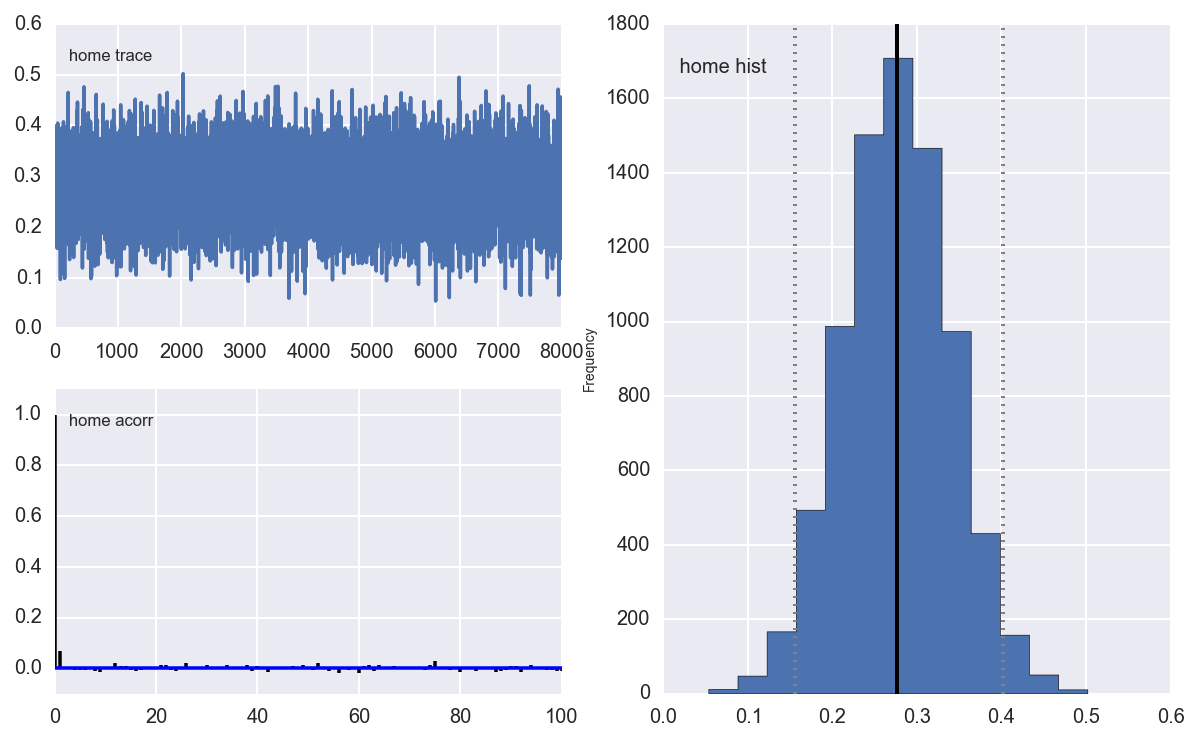The intercept also looks good, and has an HDI that doesn't include zero, indicating that including an intercept was a good idea:

In :
pymc.Matplot.plot(intercept)
Plotting intercept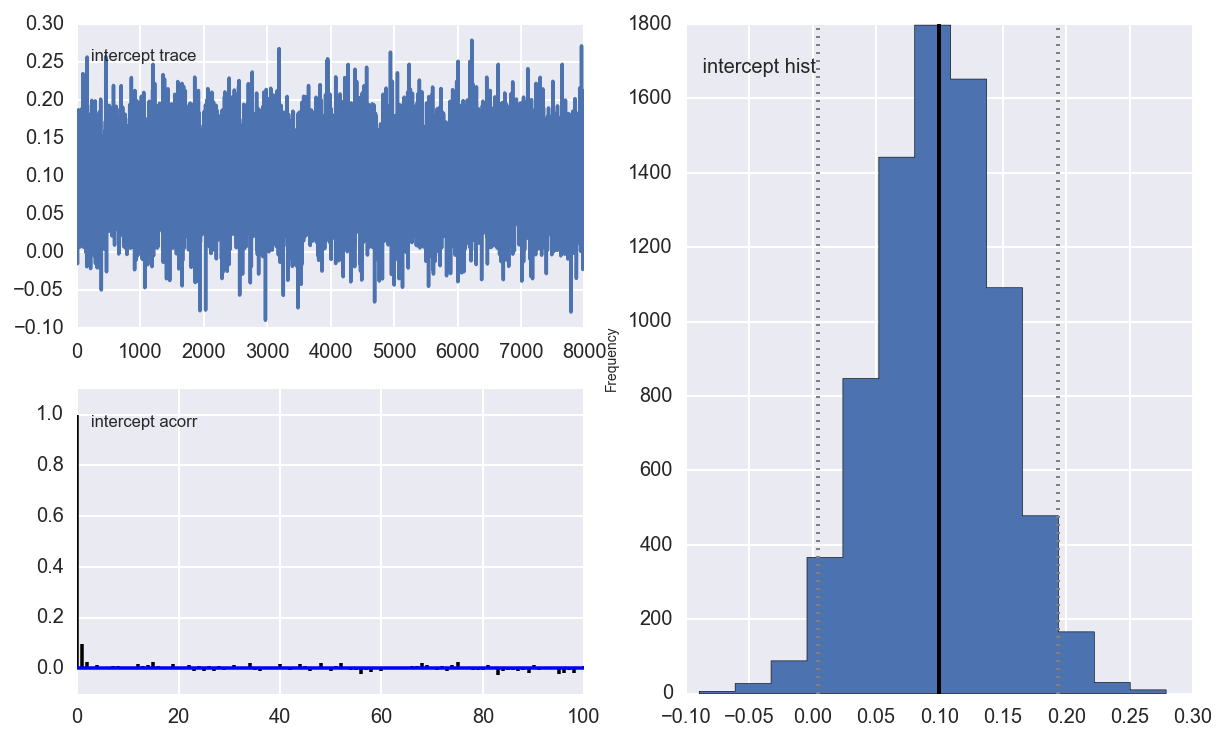The $\tau$s look ok, although there is definitely more autocorrelation in $\tau_{def}$:

In :
pymc.Matplot.plot(tau_att)
Plotting tau_att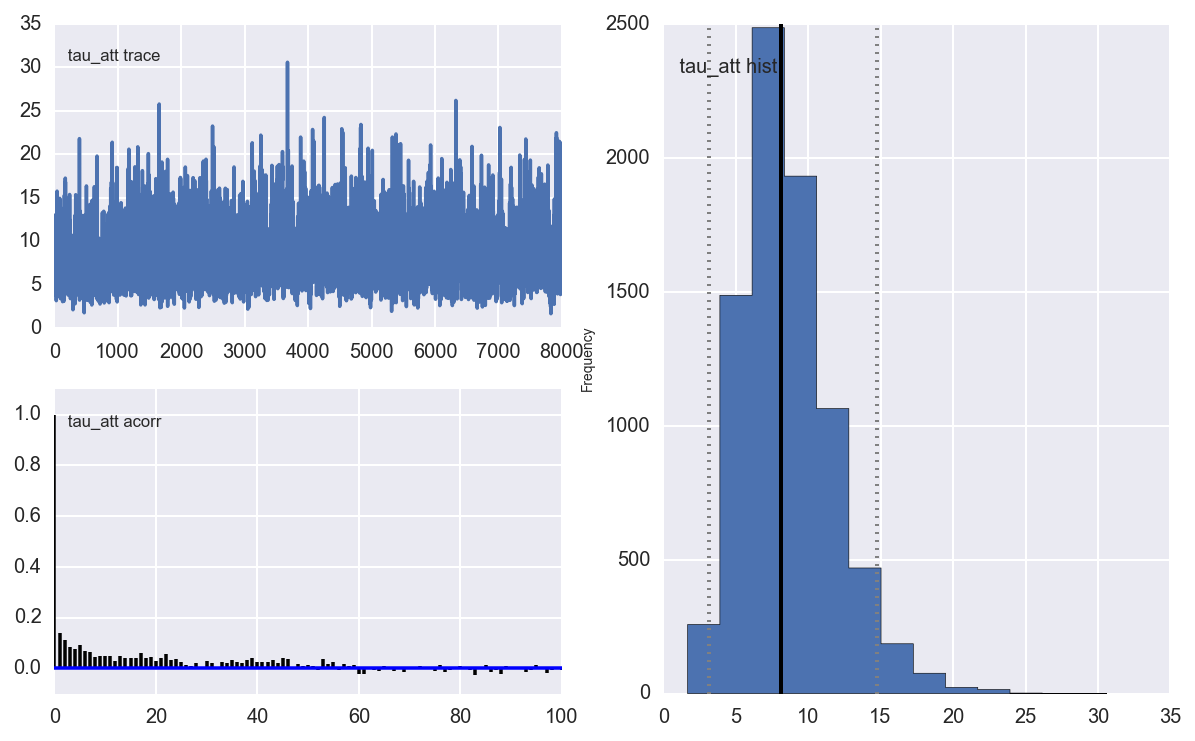In :
pymc.Matplot.plot(tau_def)
Plotting tau_def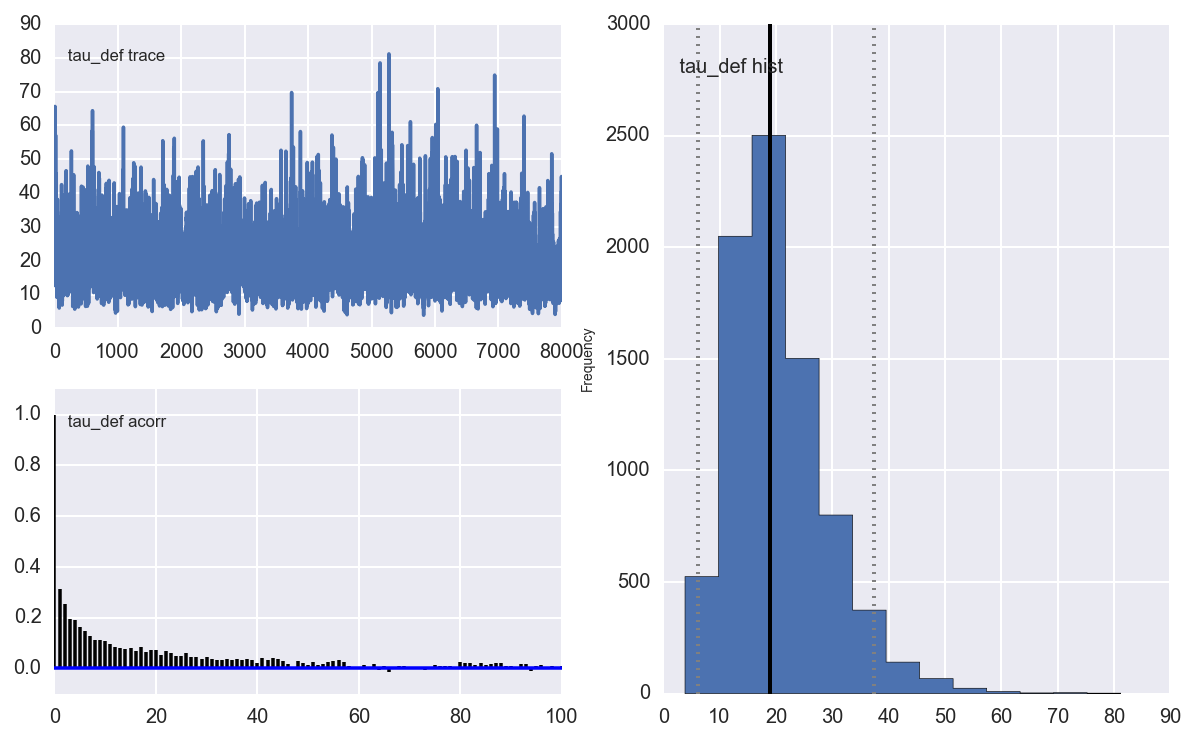Below is a subset of the atts parameters plots. To see them all, we'd just do pymc.Matplot.plot(atts)

In :
Embed = Image(CHART_DIR + 'atts.png')
Embed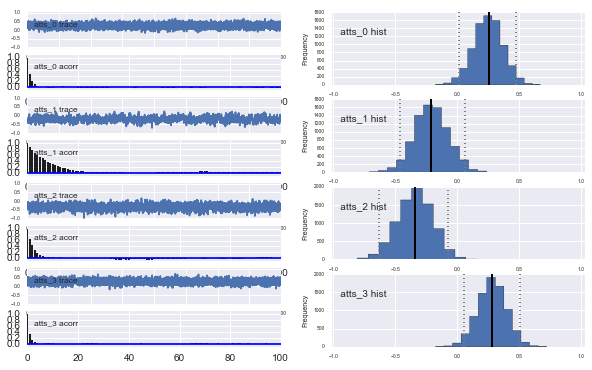Visualization¶

Let's see if we can recreate a plot (Figure 3) from their paper, of average defense effect by average attack effect. We'll add in color-coding by whether the team ended the season qualifying for the Champion's League (top 4), Europa League (5 & 6), or was relegated (bottom 3).

In :
observed_season = DATA_DIR + 'premier_league_13_14_table.csv'
df_observed.loc[df_observed.QR.isnull(), 'QR'] = ''
In :
df_avg = pd.DataFrame({'avg_att': atts.stats()['mean'],
'avg_def': defs.stats()['mean']},
index=teams.team.values)
df_avg = pd.merge(df_avg, df_observed, left_index=True, right_on='team', how='left')

fig, ax = plt.subplots(figsize=(8,6))
for outcome in ['champs_league', 'relegation', 'europa_league', '']:
ax.plot(df_avg.avg_att[df_avg.QR == outcome],
df_avg.avg_def[df_avg.QR == outcome], 'o', label=outcome)

for label, x, y in zip(df_avg.team.values, df_avg.avg_att.values, df_avg.avg_def.values):
ax.annotate(label, xy=(x,y), xytext = (-5,5), textcoords = 'offset points')
ax.set_title('Attack vs Defense avg effect: 13-14 Premier League')
ax.set_xlabel('Avg attack effect')
ax.set_ylabel('Avg defense effect')
ax.legend()
Out:
<matplotlib.legend.Legend at 0x115cd1c90>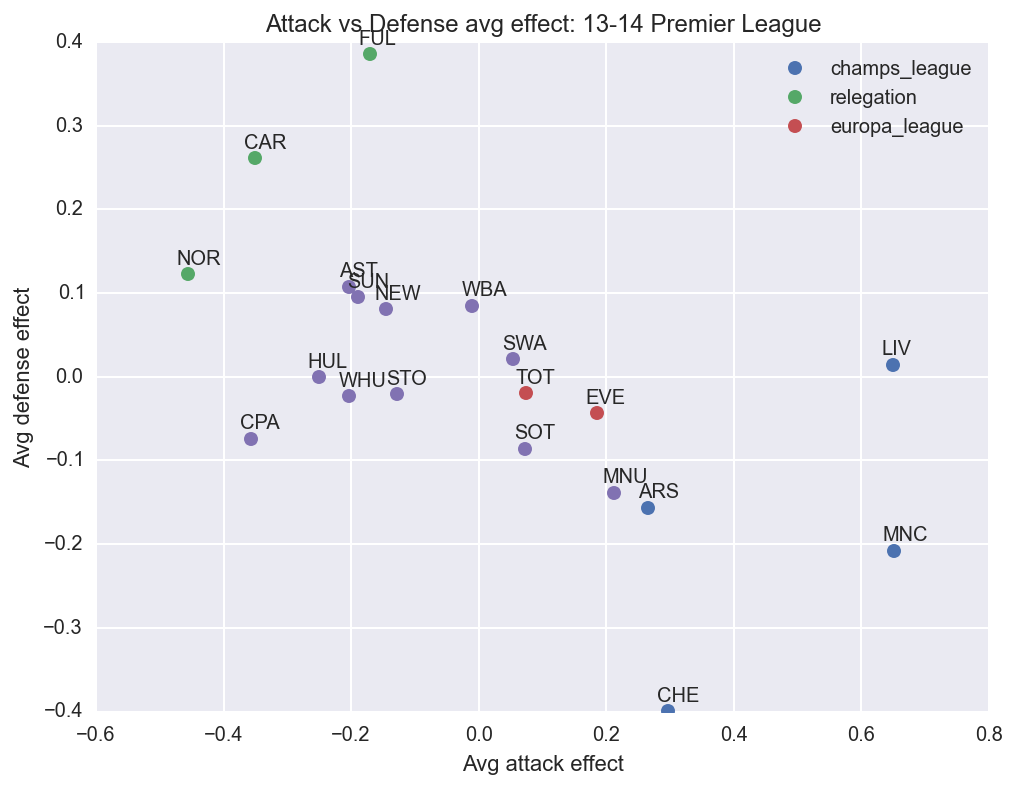So as you would expect, the top teams are in the lower right side of the chart, indicating a positive attack effect and a negative defense effect. Interestingly, Manchester United (MNU) appears to have both a stronger attack and a stronger defense than two teams that finished above it in the table, Everton (EVE) and Tottenham (TOT). But we're just looking at posterior means here, and we ought to take advantage of the fact that we can quantify our posterior uncertainty around these parameters. Let's look at the Highest Posterior Density intervals for the attack parameters.

In :
df_hpd = pd.DataFrame(atts.stats()['95% HPD interval'],
columns=['hpd_low', 'hpd_high'],
index=teams.team.values)
df_median = pd.DataFrame(atts.stats()['quantiles'],
columns=['hpd_median'],
index=teams.team.values)
df_hpd = df_hpd.join(df_median)
df_hpd['relative_lower'] = df_hpd.hpd_median - df_hpd.hpd_low
df_hpd['relative_upper'] = df_hpd.hpd_high - df_hpd.hpd_median
df_hpd = df_hpd.sort_index(by='hpd_median')
df_hpd = df_hpd.reset_index()
df_hpd['x'] = df_hpd.index + .5

fig, axs = plt.subplots(figsize=(10,4))
axs.errorbar(df_hpd.x, df_hpd.hpd_median,
yerr=(df_hpd[['relative_lower', 'relative_upper']].values).T,
fmt='o')
axs.set_title('HPD of Attack Strength, by Team')
axs.set_xlabel('Team')
axs.set_ylabel('Posterior Attack Strength')
_= axs.set_xticks(df_hpd.index + .5)
_= axs.set_xticklabels(df_hpd['index'].values, rotation=45)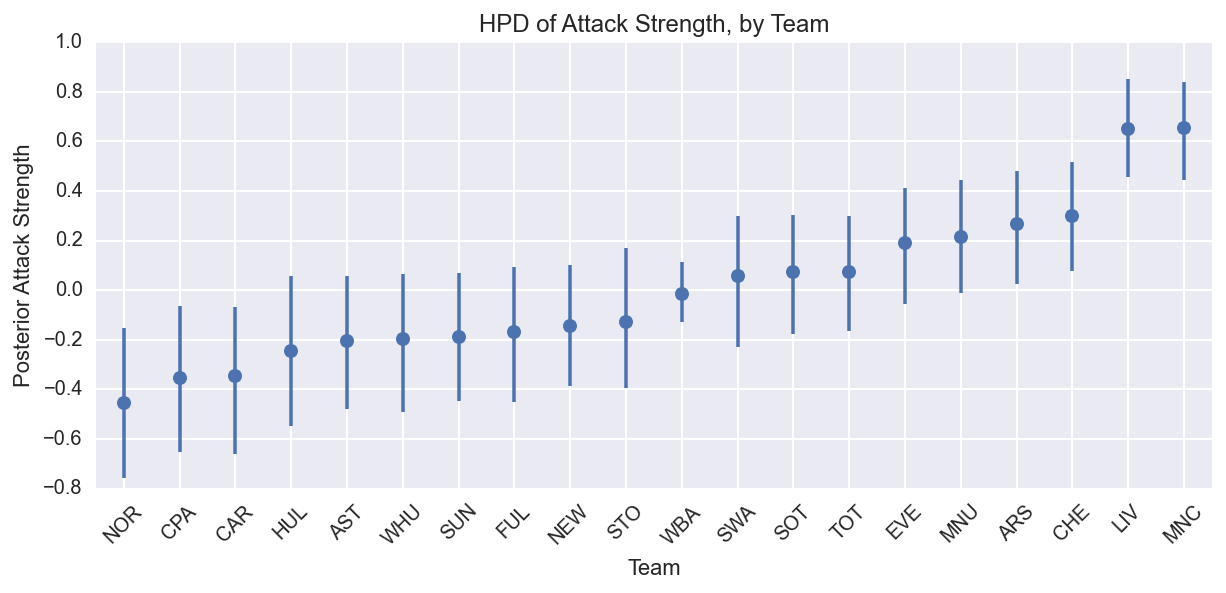Note that the intervals for MNU, EVE, and TOT overlap significantly. Indeed, there's a large set of teams in the middle with overlapping HPDs. We can be fairly confident, though, that Liverpool's and Man City's attack strength left the rest of the pack behind, which makes sense given that they each scored 30 more goals than Chelsea, the team with the third most goals scored. In fact, we might conclude that their attacks were even further from the rest of the pack if it weren't for shrinkage. To see what I mean, let's simulate some seasons.

Simulations¶

We can take draws from the posterior distributions of the parameters, and simulate a season or many seasons. Below is the simulation code, just so you can see how I did it.

In :
def simulate_season():
"""
Simulate a season once, using one random draw from the mcmc chain.
"""
num_samples = atts.trace().shape
draw = np.random.randint(0, num_samples)
atts_draw = pd.DataFrame({'att': atts.trace()[draw, :],})
defs_draw = pd.DataFrame({'def': defs.trace()[draw, :],})
home_draw = home.trace()[draw]
intercept_draw = intercept.trace()[draw]
season = df.copy()
season = pd.merge(season, atts_draw, left_on='i_home', right_index=True)
season = pd.merge(season, defs_draw, left_on='i_home', right_index=True)
season = season.rename(columns = {'att': 'att_home', 'def': 'def_home'})
season = pd.merge(season, atts_draw, left_on='i_away', right_index=True)
season = pd.merge(season, defs_draw, left_on='i_away', right_index=True)
season = season.rename(columns = {'att': 'att_away', 'def': 'def_away'})
season['home'] = home_draw
season['intercept'] = intercept_draw
season['home_theta'] = season.apply(lambda x: math.exp(x['intercept'] +
x['home'] +
x['att_home'] +
x['def_away']), axis=1)
season['away_theta'] = season.apply(lambda x: math.exp(x['intercept'] +
x['att_away'] +
x['def_home']), axis=1)
season['home_goals'] = season.apply(lambda x: np.random.poisson(x['home_theta']), axis=1)
season['away_goals'] = season.apply(lambda x: np.random.poisson(x['away_theta']), axis=1)
season['home_outcome'] = season.apply(lambda x: 'win' if x['home_goals'] > x['away_goals'] else
'loss' if x['home_goals'] < x['away_goals'] else 'draw', axis=1)
season['away_outcome'] = season.apply(lambda x: 'win' if x['home_goals'] < x['away_goals'] else
'loss' if x['home_goals'] > x['away_goals'] else 'draw', axis=1)
season = season.join(pd.get_dummies(season.home_outcome, prefix='home'))
season = season.join(pd.get_dummies(season.away_outcome, prefix='away'))
return season

def create_season_table(season):
"""
Using a season dataframe output by simulate_season(), create a summary dataframe with wins, losses, goals for, etc.

"""
g = season.groupby('i_home')
home = pd.DataFrame({'home_goals': g.home_goals.sum(),
'home_goals_against': g.away_goals.sum(),
'home_wins': g.home_win.sum(),
'home_draws': g.home_draw.sum(),
'home_losses': g.home_loss.sum()
})
g = season.groupby('i_away')
away = pd.DataFrame({'away_goals': g.away_goals.sum(),
'away_goals_against': g.home_goals.sum(),
'away_wins': g.away_win.sum(),
'away_draws': g.away_draw.sum(),
'away_losses': g.away_loss.sum()
})
df = home.join(away)
df['wins'] = df.home_wins + df.away_wins
df['draws'] = df.home_draws + df.away_draws
df['losses'] = df.home_losses + df.away_losses
df['points'] = df.wins * 3 + df.draws
df['gf'] = df.home_goals + df.away_goals
df['ga'] = df.home_goals_against + df.away_goals_against
df['gd'] = df.gf - df.ga
df = pd.merge(teams, df, left_on='i', right_index=True)
df = df.sort_index(by='points', ascending=False)
df = df.reset_index()
df['position'] = df.index + 1
df['champion'] = (df.position == 1).astype(int)
df['qualified_for_CL'] = (df.position < 5).astype(int)
df['relegated'] = (df.position > 17).astype(int)
return df

def simulate_seasons(n=100):
dfs = []
for i in range(n):
s = simulate_season()
t = create_season_table(s)
t['iteration'] = i
dfs.append(t)
return pd.concat(dfs, ignore_index=True)

Let's now simulate a thousand seasons.

In :
simuls = simulate_seasons(1000)

How did Man City do across our 1000 simulations? Below is a histogram of the number of points they had at season's end:

In :
ax = simuls.points[simuls.team == 'MNC'].hist(figsize=(7,5))
median = simuls.points[simuls.team == 'MNC'].median()
ax.set_title('Man City: 2013-14 points, 1000 simulations')
ax.plot([median, median], ax.get_ylim())
plt.annotate('Median: %s' % median, xy=(median + 1, ax.get_ylim()-10))
Out:
<matplotlib.text.Annotation at 0x1152d9d90>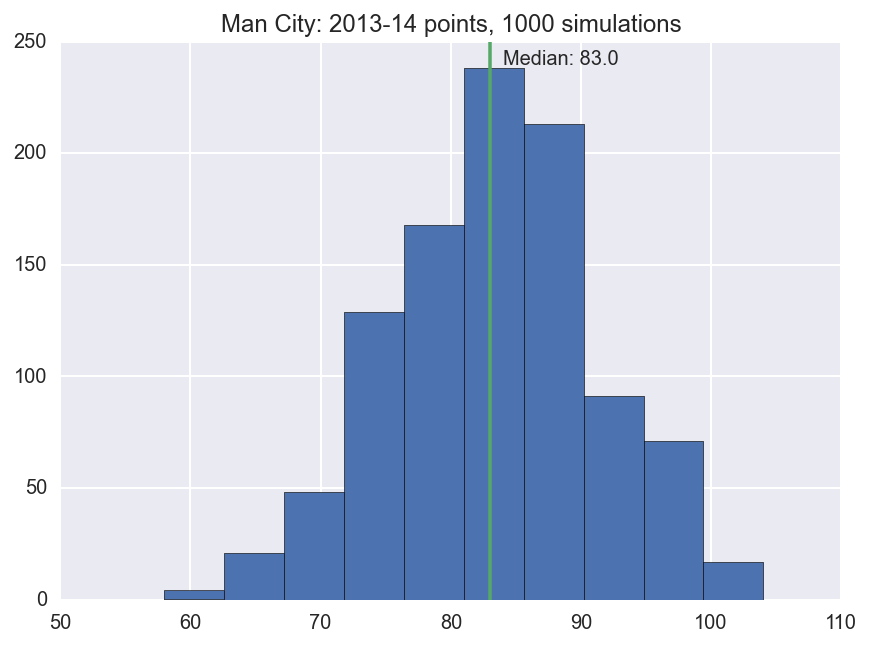And how many goals they scored:

In :
ax = simuls.gf[simuls.team == 'MNC'].hist(figsize=(7,5))
median = simuls.gf[simuls.team == 'MNC'].median()
ax.set_title('Man City: 2013-14 goals for, 1000 simulations')
ax.plot([median, median], ax.get_ylim())
plt.annotate('Median: %s' % median, xy=(median + 1, ax.get_ylim()-10))
Out:
<matplotlib.text.Annotation at 0x114a9e610>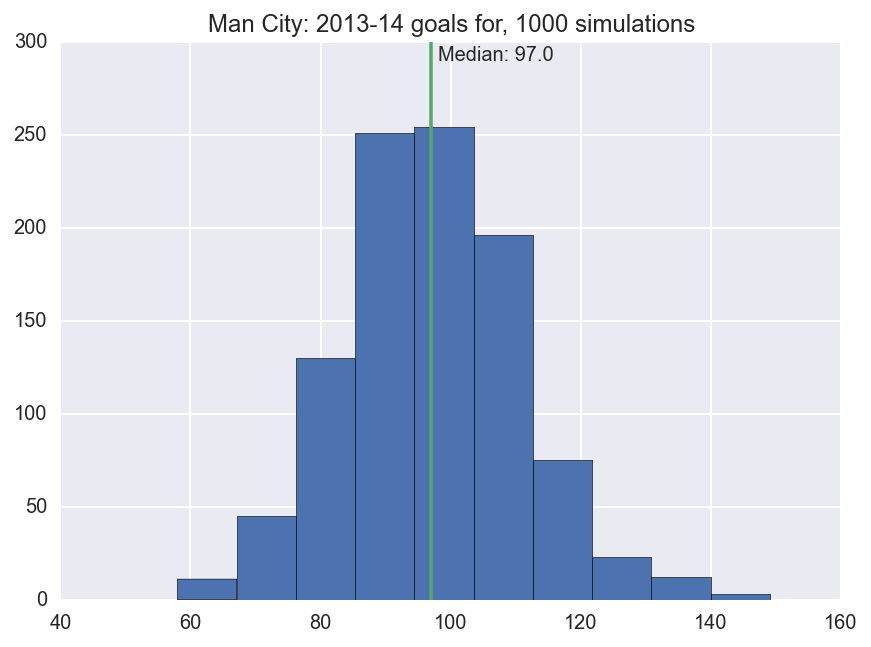Note that the median simulation has Man city scorting 95 goals, 7 fewer than they actually scored last season. I wonder if something similar is going on for other teams. Let's check.

In :
g = simuls.groupby('team')
season_hdis = pd.DataFrame({'points_lower': g.points.quantile(.05),
'points_upper': g.points.quantile(.95),
'goals_for_lower': g.gf.quantile(.05),
'goals_for_median': g.gf.median(),
'goals_for_upper': g.gf.quantile(.95),
'goals_against_lower': g.ga.quantile(.05),
'goals_against_upper': g.ga.quantile(.95),
})
season_hdis = pd.merge(season_hdis, df_observed, left_index=True, right_on='team')
column_order = ['team', 'points_lower', 'Pts', 'points_upper',
'goals_for_lower', 'GF', 'goals_for_median', 'goals_for_upper',
'goals_against_lower', 'GA', 'goals_against_upper',]
season_hdis = season_hdis[column_order]
season_hdis['relative_goals_upper'] = season_hdis.goals_for_upper - season_hdis.goals_for_median
season_hdis['relative_goals_lower'] = season_hdis.goals_for_median - season_hdis.goals_for_lower
season_hdis = season_hdis.sort_index(by='GF')
season_hdis = season_hdis.reset_index()
season_hdis['x'] = season_hdis.index + .5
season_hdis

fig, axs = plt.subplots(figsize=(10,6))
axs.scatter(season_hdis.x, season_hdis.GF, c=sns.palettes.color_palette(), zorder = 10, label='Actual Goals For')
axs.errorbar(season_hdis.x, season_hdis.goals_for_median,
yerr=(season_hdis[['relative_goals_lower', 'relative_goals_upper']].values).T,
fmt='s', c=sns.palettes.color_palette(), label='Simulations')
axs.set_title('Actual Goals For, and 90% Interval from Simulations, by Team')
axs.set_xlabel('Team')
axs.set_ylabel('Goals Scored')
axs.set_xlim(0, 20)
axs.legend()
_= axs.set_xticks(season_hdis.index + .5)
_= axs.set_xticklabels(season_hdis['team'].values, rotation=45)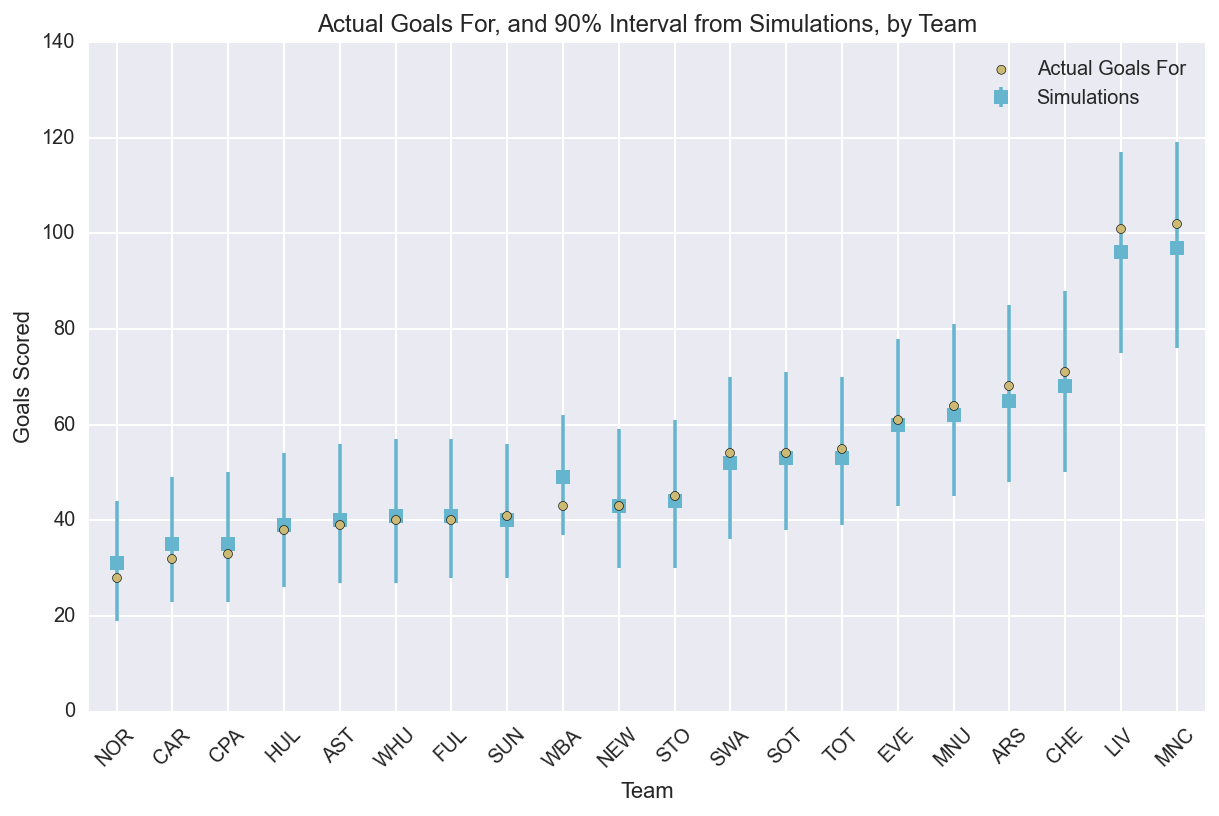Indeed, for high-scoring teams, the observed goals scored falls in the upper end of the distribution from our simulations, and the opposite is true (but to a lesser extent) for the lower-scoring teams. This is shrinkage in action.

Shrinkage¶

Recall that we defined the attack parameters as being exchangable: $att_{t} \sim Normal(0, \tau_{att})$. Shrinkage is when parameters are pulled toward their group mean; in this case, the team-specific attack parameters are pulled toward zero. We can see this visually in the chart above: the blue squares (simulation medians) are all closer to the mean than the observations (yellow circles).

One way to think about shrinkage in this model is that we're using the data on all the teams to inform our inference on each individual team. We're using the fact that most teams scored 40-60 goals in the season to infer that Liverpool's and Man City's 100+ goal seasons were a bit lucky.

In the Baio and Blangiardo paper, they address the shrinkage by creating a more complicated model:

The model of §2 assumes that all the attack and defense propensities be drawn by a common process, characterised by the common vector of hyper- parameters ($\mu_{att}$, $\tau_{att}$, $\mu_{def}$, $\tau_{def}$); clearly, this might be not sufficient to capture the presence of different quality in the teams, therefore producing overshrinkage, with the effect of: a) penalising extremely good teams; and b) overestimate the performance of poor teams. One possible way to avoid this problem is to introduce a more complicated structure for the parameters of the model, in order to allow for three different generating mechanism, one for the top teams, one for the mid-table teams, and one for the bottom-table teams.

That makes a lot of sense. There does seem to be a top tier of teams that is just a leg above the rest. (I tried implementing their more complicated model, but I couldn't get it to converge nicely).

I had some trouble wrapping my mind around how we decide when shrinkage is a problem - i.e. how do we choose between the simple model with some shrinkage and the more complicated model - so I emailed John Kruschke (author of the puppy book), who replied:

• There's nothing inherently good or bad about shrinkage in hierarchical models. Shrinkage is just the mathematical consequence of the model choice. Just as you can choose to model a trend with a line or a quadratic or an exponential, you choose to model data with various hierarchies of parameters. We choose a model to achieve both descriptive adequacy and theoretical meaningfulness. If a particular choice of hierarchical model seems to produce too much shrinkage in some of the parameters, it means that somehow the hierarchy did not capture your prior knowledge because the posterior seems to violate your prior knowledge in ways unjustifiable from the data.

• Hierarchical models are supposed to express prior knowledge about how subsets of data are related. If the various subsets can truly be thought of as representing a higher level, then it makes perfect sense to put the subsets under a hierarchy and use all the the subsets to mutually inform the higher level and simultaneously constrain the lower-level estimates. The higher level estimate essentially constitutes a prior for each of the lower-level parameters.

So in this case, is the simple model adequate? On the one hand, the observed shrinkage isn't too egregious, and the model has allowed Liverpool and Man City to be in an attacking class of their own. On the other hand, we have some prior knowledge in favor of there being different tiers of team strength: we can look at prior seasons, or at the wage bills. The chart below is from 2012-2013 (select 'Wage Bill' from the dropdown), but illustrates that there are at least two tiers when it comes to team wages: those spending more than £130m, and those spending less than £100m.

In :
HTML('<iframe src="http://cf.datawrapper.de/USTan/2/" frameborder="0" allowtransparency="true" allowfullscreen webkitallowfullscreen mozallowfullscreen oallowfullscreen msallowfullscreen width="460" height="700"></iframe>')
Out:

I don't think there's a correct/incorrect answer here. For a case that's more cut-and-dry, see this post by John Kruschke, in which he models baseball batting averages. Because batting averages vary significantly by position - pitchers aren't hired for their batting ability - it makes sense to model batting averages as coming from position-specific distributions.

Come on You Spurs¶

Lastly, just for fun, let's see in what percentage of simulations each team finished in the top 4, qualifying for the Champions League:

In :
g = simuls.groupby('team')
df_champs = pd.DataFrame({'percent_champs': g.champion.mean(),
'percent_CL': g.qualified_for_CL.mean()})
df_champs = df_champs.sort_index(by='percent_CL')
df_champs = df_champs[df_champs.percent_CL > .05]
df_champs = df_champs.reset_index()

fig, ax = plt.subplots(figsize=(8,6))
ax.barh(df_champs.index.values, df_champs.percent_CL.values)

for i, row in df_champs.iterrows():
label = "{0:.1f}%".format(100 * row['percent_CL'])
ax.annotate(label, xy=(row['percent_CL'], i), xytext = (3, 10), textcoords = 'offset points')
ax.set_ylabel('Team')
ax.set_title('% of Simulated Seasons In Which Team Finished in Top 4')
_= ax.set_yticks(df_champs.index + .5)
_= ax.set_yticklabels(df_champs['team'].values)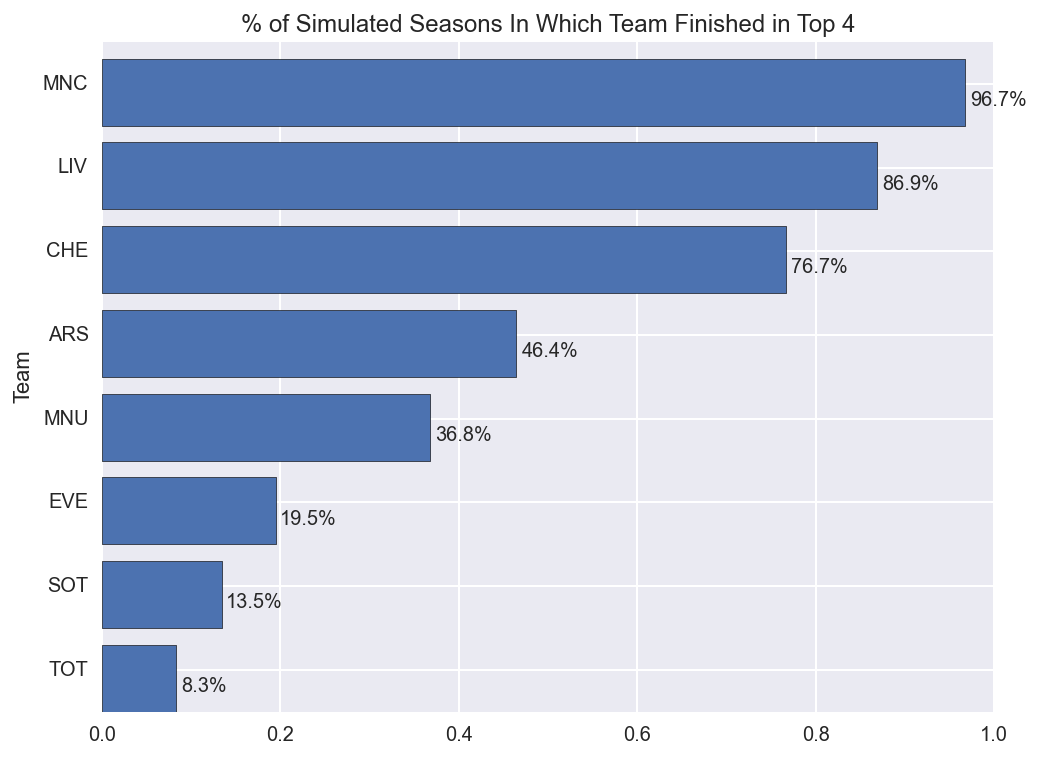In ~8.3% of alternative universes, Tottenham finished in the top 4. If the me from that universe is reading this and wants to swap, please contact me.